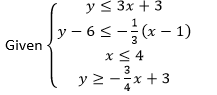# Polygons and Algebraic Relationships

## Objective

Calculate and justify the area and perimeter of polygons on the coordinate plane given a system of inequalities.

## Common Core Standards

### Core Standards

?

• G.GPE.B.4 — Use coordinates to prove simple geometric theorems algebraically. For example, prove or disprove that a figure defined by four given points in the coordinate plane is a rectangle; prove or disprove that the point (1, √3) lies on the circle centered at the origin and containing the point (0, 2).

• G.GPE.B.7 — Use coordinates to compute perimeters of polygons and areas of triangles and rectangles, e.g., using the distance formula. Modeling is best interpreted not as a collection of isolated topics but in relation to other standards. Making mathematical models is a Standard for Mathematical Practice, and specific modeling standards appear throughout the high school standards indicated by a star symbol (★). The star symbol sometimes appears on the heading for a group of standards; in that case, it should be understood to apply to all standards in that group.

• A.REI.D.12 — Graph the solutions to a linear inequality in two variables as a half-plane (excluding the boundary in the case of a strict inequality), and graph the solution set to a system of linear inequalities in two variables as the intersection of the corresponding half-planes.

?

• 6.G.A.3

• 8.G.B.8

## Criteria for Success

?

1. Create a polygon by graphing a system of inequalities.
2. Prove a given system of inequalities creates a specific polygon.
3. Find the area and perimeter given a system of inequalities.

?

## Anchor Problems

?

### Problem 1

${\mathrm{Given}\left\{\begin{matrix}y \leq4 \\ y \geq0 \\ y \leq 2x \\ y + 8 \geq 2x \end{matrix}\right.}$

1. Graph the system of inequalities.
2. Prove that the polygon created is a parallelogram.

### Problem 2

${\mathrm{Given}\left\{\begin{matrix}y \leq -3x+8 \\ y \leq x \\ 3y \geq x-6 \end{matrix}\right.}$

1. Show that the triangle created is a right triangle.
2. Find the area of the triangle.
3. Find the perimeter of the triangle. Round your answer to the nearest tenth.

## Problem Set

?

The following resources include problems and activities aligned to the objective of the lesson that can be used to create your own problem set.

• Include problems where students are given a system of inequalities and asked to find the area and perimeter of a polygon.
• Include problems where students are given a system of inequalities and must show that the polygon created is a parallelogram, rectangle, rhombus, and/or square.